# Using Aggregate Combinators in ClickHouseDenys Golotiuk
Feb 8, 2023ClickHouse supports not only standard aggregate functions but also a lot of more advanced ones to cover most analytical use cases. Along with aggregate functions, ClickHouse provides aggregate combinators, which are a powerful extension to the querying capabilities and can address a massive number of requirements.

Combinators allow extending and mixing aggregations to address a wide range of data structures. This capability will enable us to adapt queries instead of tables to answer even the most complex questions.

In this blog post, we explore Aggregate Combinators and how the can potentially simplify your queries and avoid the need to make structural changes to your data.

## How to use combinators

To use a combinator, we have to do two things. First, choose an aggregate function we want to use; let's say we want a `sum()` function. Second, pick a combinator needed for our case; let's say we need an `If` combinator. To use this in a query, we add the combinator to the function name:

```
SELECT sumIf(...)

```

An even more useful feature is that we can combine any number of combinators in a single function:

```
SELECT sumArrayIf(...)

```

Here, we've combined the `sum()` function with the `Array` and `If` combinators: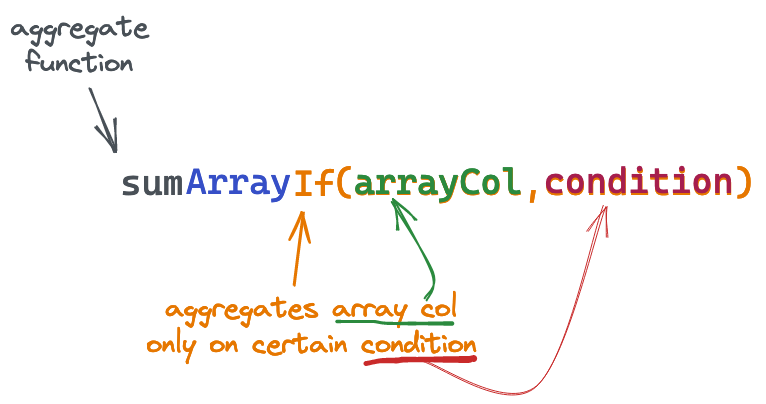This particular example would allow us to conditionally sum the contents of an array column.

Let's explore some practical cases where combinators can be used.

Sometimes, we need to aggregate data based on specific conditions. Instead of using a `WHERE` clause for this, we can use `If` combinator and specify conditions as a last argument of the combined function: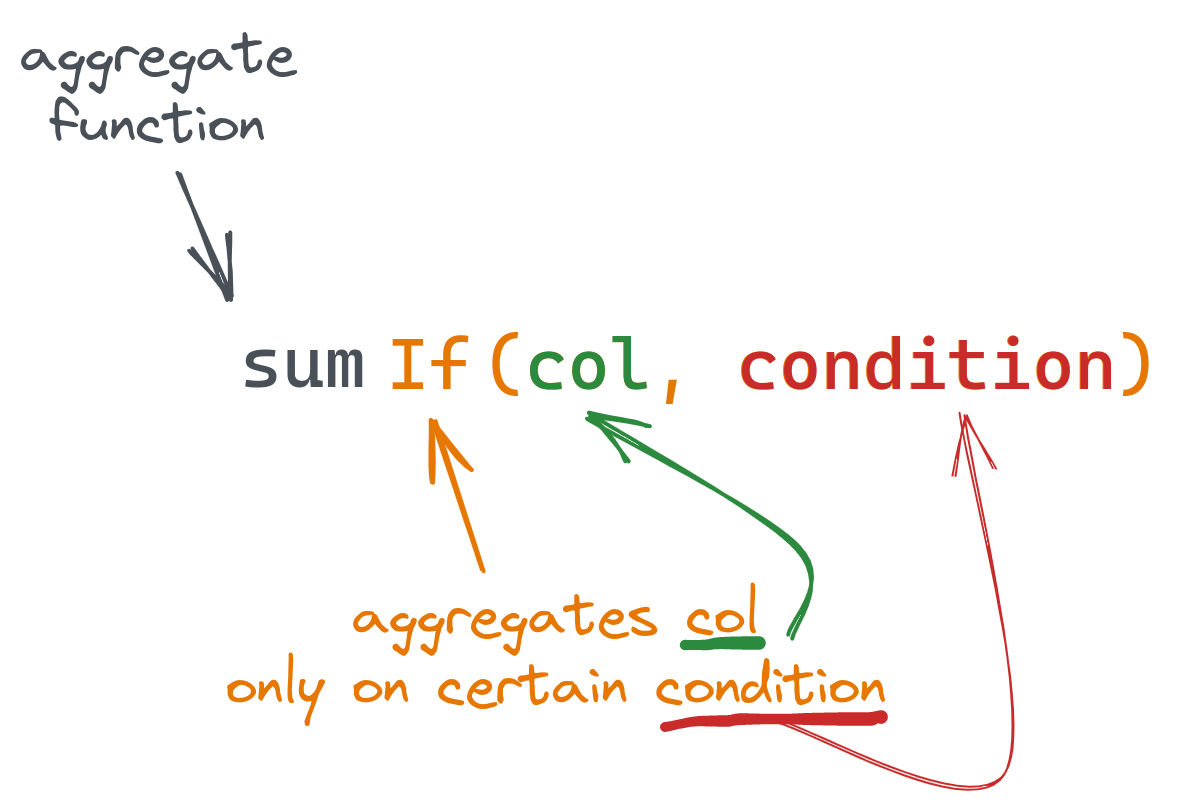Suppose we have a table with user payments of the following structure (populated with a sample data):

```
CREATE TABLE payments
(
`total_amount` Float,
`status` ENUM('declined', 'confirmed'),
`create_time` DateTime,
`confirm_time` DateTime
)
ENGINE = MergeTree
ORDER BY (status, create_time)

```

Let's say we want to get the total amount spent, but only when the payment was confirmed i.e. `status="confirmed"`:

```
SELECT sumIf(total_amount, status = 'confirmed') FROM payments

┌─sumIf(total_amount, equals(status, 'declined'))─┐
│                               10780.18000793457 │
└─────────────────────────────────────────────────┘

```

We can use the same syntax for the condition as for `WHERE` clauses. Let's get the total amount of confirmed payments, but when `confirm_time` is later than `create_time` by 1 minute:

```
SELECT sumIf(total_amount, (status = 'confirmed') AND (confirm_time > (create_time + toIntervalMinute(1)))) AS confirmed_and_checked
FROM payments

┌─confirmed_and_checked─┐
│     11195.98991394043 │
└───────────────────────┘

```

The principal advantage of using the conditional `If`, over a standard `WHERE` clause, is the ability to compute multiple sums for different clauses. We can also use any available aggregate function with combinators, like `countIf()`, `avgIf()` or `quantileIf()` - any. Combing these capabilities we can aggregate on multiple conditions and functions within a single request:

```
SELECT
countIf((status = 'confirmed') AND (confirm_time > (create_time + toIntervalMinute(1)))) AS num_confirmed_checked,
sumIf(total_amount, (status = 'confirmed') AND (confirm_time > (create_time + toIntervalMinute(1)))) AS confirmed_checked_amount,
countIf(status = 'declined') AS num_declined,
sumIf(total_amount, status = 'declined') AS dec_amount,
avgIf(total_amount, status = 'declined') AS dec_average
FROM payments

┌─num_confirmed_checked─┬─confirmed_checked_amount─┬─num_declined─┬────────dec_amount─┬───────dec_average─┐
│                    39 │        11195.98991394043 │           50 │ 10780.18000793457 │ 215.6036001586914 │
└───────────────────────┴──────────────────────────┴──────────────┴───────────────────┴───────────────────┘

```

## Aggregating on unique entries only

It's a common case to calculate the number of unique entries. ClickHouse has several ways to do this using either `COUNT(DISTINCT col)` (the same as uniqExact) or the`uniq()` when an estimated (but faster) value is sufficient. Still, we might want to have unique values from a column used in different aggregate functions. The `Distinct` combinator can be used for this: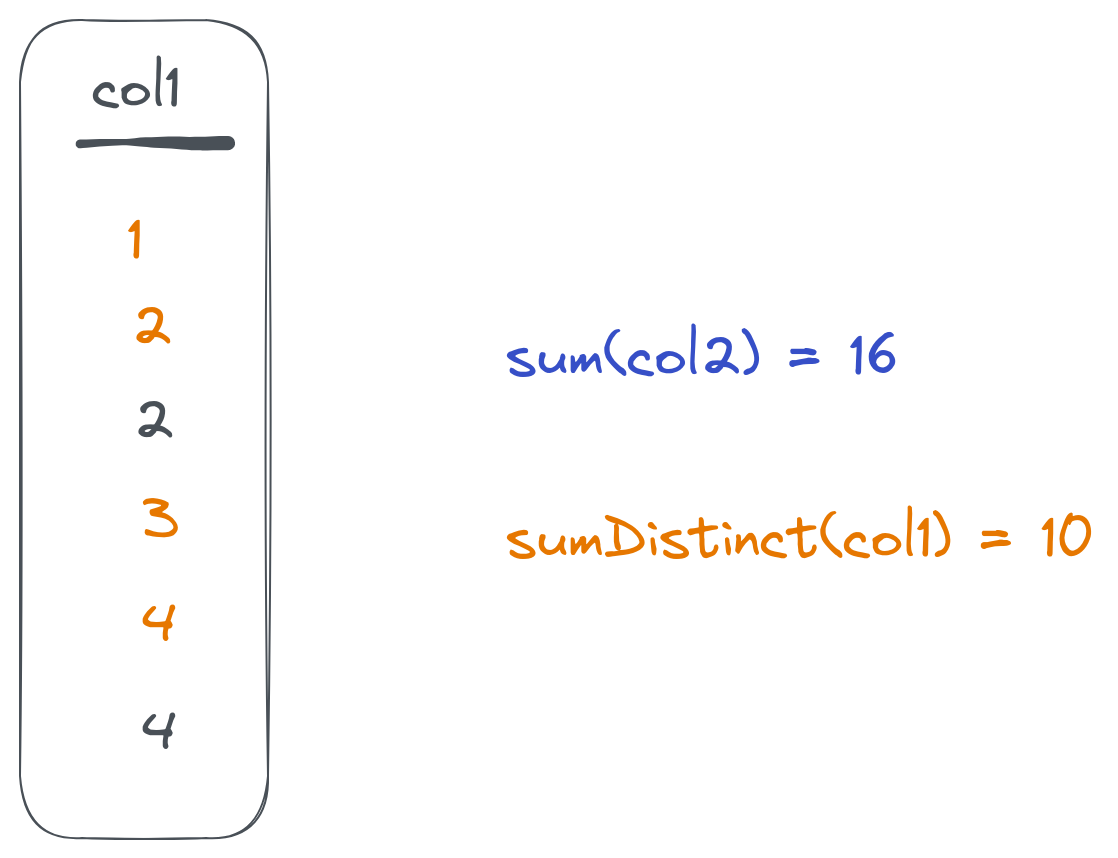Once we add `Distinct` to the aggregate function, it will ignore repeated values:

```
SELECT
countDistinct(toHour(create_time)) AS hours,
avgDistinct(toHour(create_time)) AS avg_hour,
avg(toHour(create_time)) AS avg_hour_all
FROM payments

┌─hours─┬─avg_hour─┬─avg_hour_all─┐
│     2 │     13.5 │        13.74 │
└───────┴──────────┴──────────────┘

```

Here, `avg_hour` will be calculated based on the two distinct values only, while `avg_hour_all` will be calculated based on all `100` records in the table.

### Combining `Distinct` and `If`

As combinators can be combined together, we can use both previous combinators with an `avgDistinctIf` function to address more advanced logic:

```
SELECT avgDistinctIf(toHour(create_time), total_amount > 400) AS avg_hour
FROM payments

┌─avg_hour─┐
│       13 │
└──────────┘

```

This will calculate the average on distinct hour values for records with a `total_amount` value of more than `400`.

## Splitting data into groups before aggregating

Instead of min/max analysis, we might want to split our data into groups and calculate figures for each group separately. This can be solved using the `Resample` combinator.

It takes a column, range (start/stop), and a step that you want to split data on. It then returns an aggregate value for each group: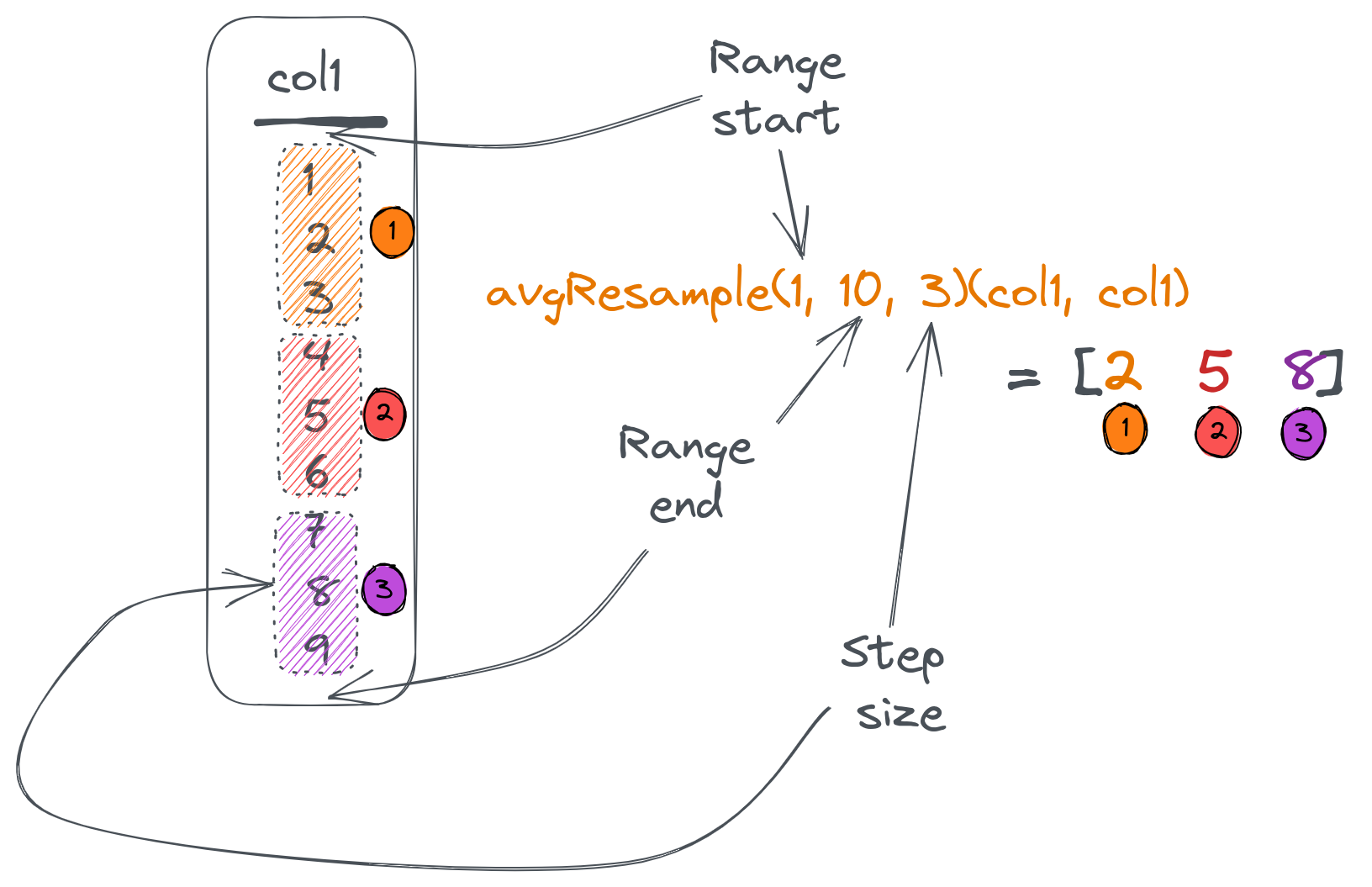Suppose we want to split our `payments` table data based on the `total_amount` from `0` (which is the minimum) to `500` (which is the maximum) with a step of `100`. Then, we want to know how many entries there are in each group as well as the groups average total:

```
SELECT
countResample(0, 500, 100)(toInt16(total_amount)) AS group_entries,
avgResample(0, 500, 100)(total_amount, toInt16(total_amount)) AS group_totals
FROM payments
FORMAT Vertical

Row 1:
──────
group_entries: [21,20,24,31,4]
group_totals:  [50.21238123802912,157.32600135803222,246.1433334350586,356.2583834740423,415.2425003051758]

```

Here, the `countResample()` function counts the number of entries in each group, and an `avgResample()` function calculates an average of the `total_amount` for each group. `Resample` combinator accepts column name to split based on as a last argument to the combined function.

Note that the `countResample()` function has only one argument (since `count()` doesn't require arguments at all) and `avgResample()` has two arguments (the first one is the column to calculate average values for). Finally, we had to use `toInt16` to convert `total_amount` to an integer since a `Resample` combinator requires this.

To get the `Resample()` combinators output in a table layout, we can use `arrayZip()` and `arrayJoin()` functions:

```
SELECT
round(tp.2, 2) AS avg_total,
tp.1 AS entries
FROM
(
SELECT
arrayJoin(arrayZip(countResample(0, 500, 100)(toInt16(total_amount)),
avgResample(0, 500, 100)(total_amount, toInt16(total_amount)))) AS tp
FROM payments
)

┌─avg_total─┬─entries─┐
│     50.21 │      21 │
│    157.33 │      20 │
│    246.14 │      24 │
│    356.26 │      31 │
│    415.24 │       4 │
└───────────┴─────────┘

```

Here, we combine corresponding values from 2 arrays into tuples and unfold the resulting array into a table using an `arrayJoin()` function:

## Controlling aggregate values for empty results

Aggregate functions react differently to cases when the resulting set contains no data. For example, `count()` will return `0` while `avg()` will produce a `nan` value.

We can control this behaviour using the `OrDefault()` and `OrNull()` combinators. Both changes a returned value of an aggregate function used in case of an empty dataset:

• `OrDefault()` will return a default value of the function instead of `nan`,
• `OrNull()` will return `NULL` (and will also change the return type to `Nullable`).

Consider the following example:

```
SELECT
count(),
countOrNull(),
avg(total_amount),
avgOrDefault(total_amount),
sumOrNull(total_amount)
FROM payments
WHERE total_amount > 1000

┌─count()─┬─countOrNull()─┬─avg(total_amount)─┬─avgOrDefault(total_amount)─┬─sumOrNull(total_amount)─┐
│       0 │          ᴺᵁᴸᴸ │               nan │                          0 │                    ᴺᵁᴸᴸ │
└─────────┴───────────────┴───────────────────┴────────────────────────────┴─────────────────────────┘

```

As we can see in the first column, zero rows were returned. Note how `countOrNull()` will return `NULL` instead of `0`, and `avgOrDefault()` gives `0` instead of `nan`.

### Using with other combinators

As well as all other combinators, `orNull()` and `orDefault()` can be used together with different combinators for a more advanced logic:

```
SELECT
sumIfOrNull(total_amount, status = 'declined') AS declined,
countIfDistinctOrNull(total_amount, status = 'confirmed') AS confirmed_distinct
FROM payments
WHERE total_amount > 420

┌─declined─┬─confirmed_distinct─┐
│     ᴺᵁᴸᴸ │                  1 │
└──────────┴────────────────────┘

```

We've used the `sumIfOrNull()` combined function to calculate only declined payments and return `NULL` on an empty set. The `countIfDistinctOrNull()` function counts distinct `total_amount` values but only for rows meeting the specified condition.

## Aggregating arrays

ClickHouse's Array type is popular among its users because it brings a lot of flexibility to table structures. To operate with `Array` columns efficiently, ClickHouse provides a set of array functions. To make aggregations on Array types easy, ClickHouse provides the `Array()` combinators. These apply a given aggregate function on all values from an array column instead of the array itself: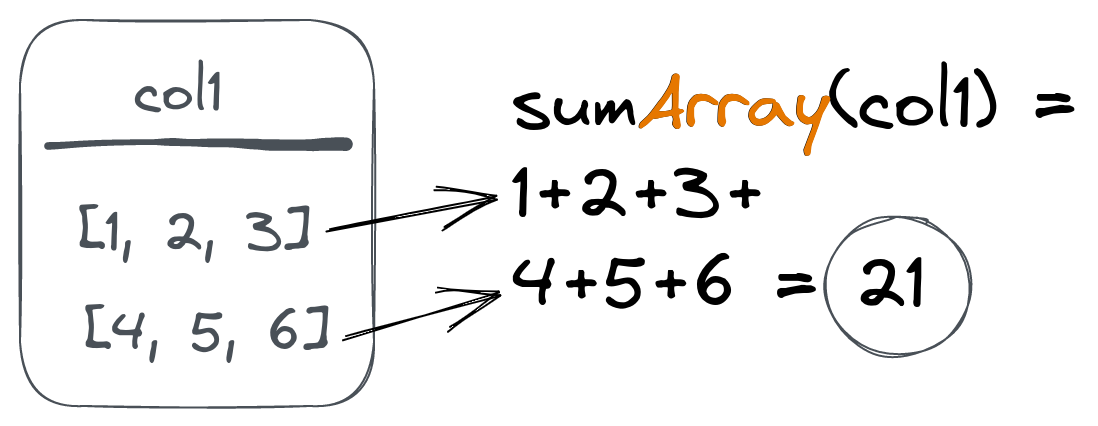Suppose we have the following table (populated with a sample data):

```
(
`time` DateTime,
`article_id` UInt32,
`sections` Array(UInt16),
`times` Array(UInt16),
`user_id` UInt32
)
ENGINE = MergeTree
ORDER BY (article_id, time)

┌────────────────time─┬─article_id─┬─sections─────────────────────┬─times────────────────────────────────┬─user_id─┐
│ 2023-01-18 23:44:17 │         10 │ [16,18,7,21,23,22,11,19,9,8] │ [82,96,294,253,292,66,44,256,222,86] │     424 │
│ 2023-01-20 22:53:00 │         10 │ [21,8]                       │ [30,176]                             │     271 │
│ 2023-01-21 03:05:19 │         10 │ [24,11,23,9]                 │ [178,177,172,105]                    │     536 │
...

```

This table is used to store article reading data for each section of the article. When a user reads an article, we save the read sections to the `sections` array column and the associated reading times to the `times` column:

Let's use the `uniqArray()` function to calculate a number of unique sections read for each article together with `avgArray()` to get an average time per section:

```
SELECT
article_id,
round(avgArray(times)) time_per_section
GROUP BY article_id

│         14 │            22 │              175 │
│         18 │            25 │              159 │
...
│         17 │            25 │              170 │
└────────────┴───────────────┴──────────────────┘

```

We can get the min and max read time across all articles using `minArray()` and `maxArray()` functions:

```
SELECT
minArray(times),
maxArray(times)

┌─minArray(times)─┬─maxArray(times)─┐
│              30 │             300 │
└─────────────────┴─────────────────┘

```

We can also get a list of read sections for each article using the `groupUniqArray()` function combined with an `Array()` combinator:

```
SELECT
article_id,
groupUniqArrayArray(sections)
GROUP BY article_id

┌─article_id─┬─groupUniqArrayArray(sections)───────────────────────────────────────┐
│         14 │ [16,13,24,8,10,3,9,19,23,14,7,25,2,1,21,18,12,17,22,4,6,5]          │
...
│         17 │ [16,11,13,8,24,10,3,9,23,19,14,7,25,20,2,1,15,21,6,5,12,22,4,17,18] │
└────────────┴─────────────────────────────────────────────────────────────────────┘

```

Another popular function is `any()`, which returns any column value under aggregation, and can also be combined `Array`:

```
SELECT
article_id,
anyArray(sections)
GROUP BY article_id

┌─article_id─┬─anyArray(sections)─┐
│         14 │                 19 │
│         18 │                  6 │
│         19 │                 25 │
│         15 │                 15 │
│         20 │                  1 │
│         16 │                 23 │
│         12 │                 16 │
│         11 │                  2 │
│         10 │                 16 │
│         13 │                  9 │
│         17 │                 20 │
└────────────┴────────────────────┘

```

### Using `Array` with other combinators

The `Array` combinator can be used together with any other combinator:

```
SELECT
article_id,
sumArrayIfOrNull(times, length(sections) > 8)
GROUP BY article_id

┌─article_id─┬─sumArrayOrNullIf(times, greater(length(sections), 8))─┐
│         14 │                                                  4779 │
│         18 │                                                  3001 │
│         19 │                                                  NULL │
...
│         17 │                                                 14424 │
└────────────┴───────────────────────────────────────────────────────┘

```

We have used the `sumArrayIfOrNull()` function to calculate the total times for articles where more than eight sections were read. Note that `NULL` is returned for articles with zero cases of more than eight sections read because we've also used the `OrNull()` combinator.

We can address even more advanced cases if we use array functions along with combinators:

```
SELECT
article_id,
countArray(arrayFilter(x -> (x > 120), times)) AS sections_engaged
GROUP BY article_id

┌─article_id─┬─sections_engaged─┐
│         14 │               26 │
│         18 │               44 │
...
│         17 │               98 │
└────────────┴──────────────────┘

```

Here, we first filter the `times` array using an `arrayFilter` function to remove all values under 120 seconds. Then, we use `countArray` to calculate filtered times (which means engaged reads in our case) for each article.

### Aggregating maps

Another powerful type available in ClickHouse is the Map. Like arrays, we can use `Map()` combinator to apply aggregations to this type.

Suppose we have the following table with a `Map` column type:

```
(
`time` DateTime,
`url` String,
`params` Map(String, UInt32)
)
ENGINE = MergeTree
ORDER BY (url, time)

┌────────────────time─┬─url─┬─params───────────────────────────────┐
│ 2023-01-25 17:44:26 │ /   │ {'load_speed':100,'scroll_depth':59} │
│ 2023-01-25 17:44:37 │ /   │ {'load_speed':400,'scroll_depth':12} │
└─────────────────────┴─────┴──────────────────────────────────────┘

```

We can use a `Map()` combinator for the `sum()` and `avg()` functions to get total loading times and average scroll depth:

```
SELECT
avgMap(params)['scroll_depth'] AS average_scroll

│             500 │           35.5 │
└─────────────────┴────────────────┘

```

The `Map()` combinator can also be used with other combinators:

```
SELECT sumMapIf(params, url = '/404')['scroll_depth'] AS average_scroll FROM page_loads

```

## Aggregating respective array values

Another way to work with array columns is to aggregate corresponding values from two arrays. This results in another array. This can be used for vectorized data (like vectors or matrices) and is implemented via the `ForEach()` combinator: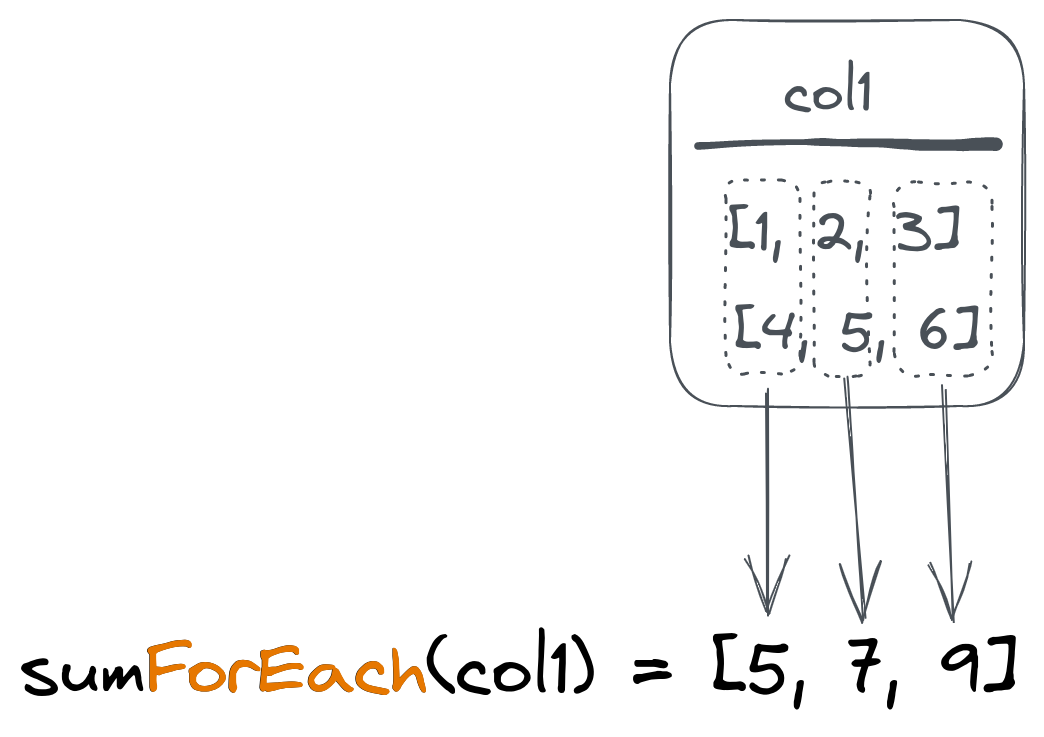Suppose we have the following table with vectors:

```
SELECT * FROM vectors

┌─title──┬─coordinates─┐
│ first  │ [1,2,3]     │
│ second │ [2,2,2]     │
│ third  │ [0,2,1]     │
└────────┴─────────────┘

```

To calculate the average coordinates array (vector), we can use an `avgForEach()` combined function:

```
SELECT avgForEach(coordinates) FROM vectors

┌─avgForEach(coordinates)─┐
│ [1,2,2]                 │
└─────────────────────────┘

```

This will ask ClickHouse to calculate an average value for the first element of all `coordinates` arrays and put it into the first element of the resulting array. Then repeat the same for the second and third elements.

And, of course, use with other combinators is also supported:

```
SELECT avgForEachIf(coordinates, title != 'second') FROM vectors

┌─avgForEachIf(coordinates, notEquals(title, 'second'))─┐
│ [0.5,2,2]                                             │
└───────────────────────────────────────────────────────┘

```

## Working with aggregation states

ClickHouse allows working with intermediate aggregation states instead of resulting values. Let's say we need to count unique values in our case, but we don't want to save the values themselves (because it takes space). In this case, we can use a `State()` combinator for the `uniq()` function to save the intermediate aggregation state and then use a `Merge()` combinator to calculate actual value:

```
SELECT uniqMerge(u)
FROM
(
SELECT uniqState(number) AS u FROM numbers(5)
UNION ALL
SELECT uniqState(number + 1) AS u FROM numbers(5)
)

┌─uniqMerge(u)─┐
│            6 │
└──────────────┘

```

Here, the first nested query will return the state for the unique count of `1...5` numbers. The second nested query returns the same for `2...6` numbers. The parent query then uses the `uniqMerge()` function to merge our states and get a count of all unique numbers we saw: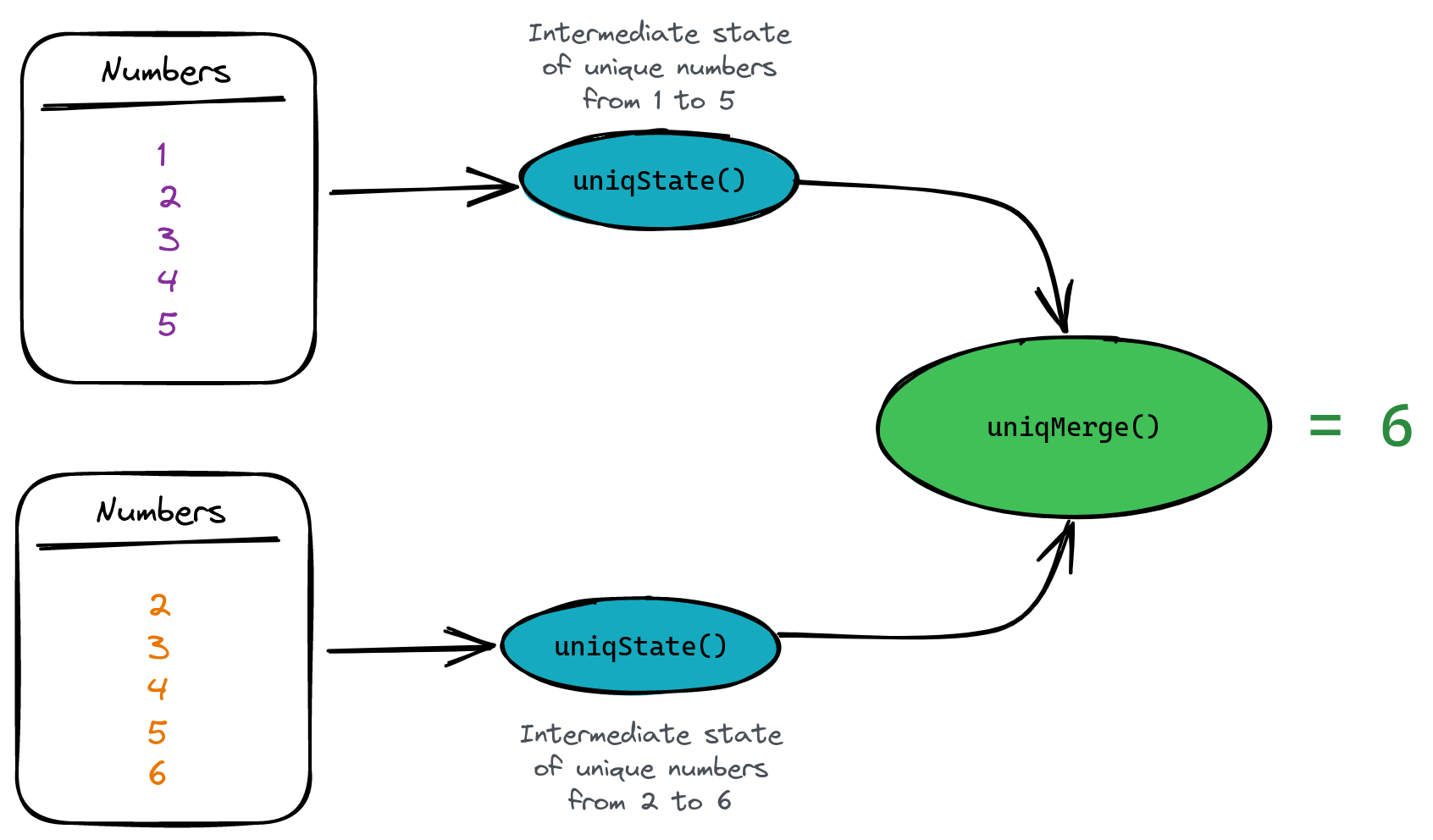Why do we want to do this? Simply because aggregate states take much less space than the original data. This is particulartly important when we want to store this state on disk. For example, `uniqState()` data takes 15 times less space than 1 million integer numbers:

```
SELECT
table,
FROM system.tables
WHERE table LIKE 'numbers%'

┌─table─────────┬─size───────┐
│ numbers       │ 3.82 MiB   │ <- we saved 1 million ints here
│ numbers_state │ 245.62 KiB │ <- we save uniqState for 1m ints here
└───────────────┴────────────┘

```

ClickHouse provides an `AggregatingMergeTree` table engine for storing aggregation states and automatically merging them on the primary key. Let's create a table to store aggregated data for daily payments from our previous examples:

```
CREATE TABLE payments_totals
(
`date` Date,
`total_amount` AggregateFunction(sum, Float)
)
ENGINE = AggregatingMergeTree
ORDER BY date

```

We've used the `AggregateFunction` type to let ClickHouse know we're going to store aggregated total states instead of scalar values. On insert, we need to use the `sumState` function to insert the aggregate state:

```
INSERT INTO payments_totals SELECT
date(create_time) AS date,
sumState(total_amount)
FROM payments
WHERE status = 'confirmed'
GROUP BY date

```

Finally, we need to use the `sumMerge()` function to fetch the resulting values:

```
┌─sumMerge(total_amount)─┐
│     12033.219916582108 │
└────────────────────────┘

```

Note that ClickHouse provides an easy way to use aggregated table engines based on materialized views. ClickHouse also provides a SimpleState combinator as an optimized version that can be used with some aggregate functions (like 'sum' or 'min').

## Summary

Aggregation function combinators bring almost limitless possibilities to analytical querying on top of any data structure in ClickHouse. We can add conditions to aggregations, apply functions to array elements or get intermediate states to store data in aggregated form but still available for querying.